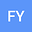loading page

Global structure and one-sign solutions for second-order Sturm-Liouville difference equation with sign-changing weight
•## Abstract

This paper is devoted to study the discrete Sturm-Liouville problem $$\left\{\begin{array}{ll} -\Delta(p(k)\Delta u(k-1))+q(k)u(k)=\lambda m(k)u(k)+f_1(k,u(k),\lambda)+f_2(k,u(k),\lambda),\ \ k\in[1,T]_Z,\\[2ex] a_0u(0)+b_0\Delta u(0)=0,\ a_1u(T)+b_1\Delta u(T)=0, \end{array}\right.$$ where $\lambda\in\mathbb{R}$ is a parameter, $f_1, f_2\in C([1,T]_Z\times\mathbb{R}^2, \mathbb{R})$, $f_1$ is not differentiable at the origin and infinity. Under some suitable assumptions on nonlinear terms, we prove the existence of unbounded continua of positive and negative solutions of this problem which bifurcate from intervals of the line of trivial solutions or from infinity, respectively.

#### Peer review status:UNDER REVIEW

08 Dec 2020Submitted to Mathematical Methods in the Applied Sciences
09 Dec 2020Assigned to Editor
09 Dec 2020Submission Checks Completed
21 Dec 2020Reviewer(s) Assigned
14 Apr 2021Review(s) Completed, Editorial Evaluation Pending